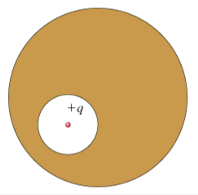# Problem: A spherical cavity is hollowed out of the interior of a neutral conducting sphere. At the center of the cavity is a point charge, of positive charge q.(a) What is the total surface charge qint on the interior surface of the conductor (i.e., on the wall of the cavity)? (b) What is the total surface charge qext on the exterior surface of the conductor?(c) What is the magnitude Eint of the electric field inside the cavity as a function of the distance r from the point charge?

###### FREE Expert Solution

(a)

The total surface charge in the conductor is zero.

q + qint = 0

93% (225 ratings)###### Problem Details

A spherical cavity is hollowed out of the interior of a neutral conducting sphere. At the center of the cavity is a point charge, of positive charge q.(a) What is the total surface charge qint on the interior surface of the conductor (i.e., on the wall of the cavity)?

(b) What is the total surface charge qext on the exterior surface of the conductor?

(c) What is the magnitude Eint of the electric field inside the cavity as a function of the distance r from the point charge?

Frequently Asked Questions

What scientific concept do you need to know in order to solve this problem?

Our tutors have indicated that to solve this problem you will need to apply the Gauss' Law concept. You can view video lessons to learn Gauss' Law. Or if you need more Gauss' Law practice, you can also practice Gauss' Law practice problems.

What professor is this problem relevant for?

Based on our data, we think this problem is relevant for Professor Kalakhety's class at Texas A & M University - Kingsville.